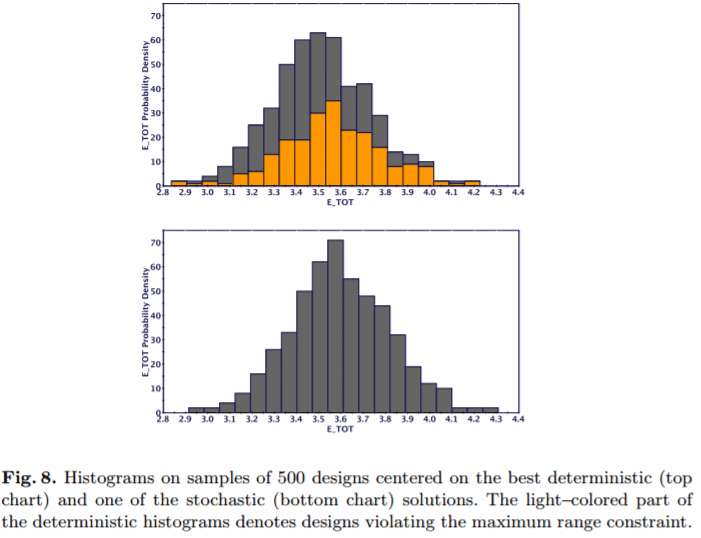# Guideline Identification for Optimization under Uncertainty through the Optimization of a Boomerang Trajectory

Author(s):
Mariapia Marchi, Enrico Rigoni, Rosario Russo, and Alberto Clarich (ESTECO)

CHALLENGE - Optimization under uncertainty (OUU) is a very important task for practitioners of engineering design optimization. The search for robust optimal solutions is intrinsically multiobjective, being formulated as the search for the optimal performance while minimizing its variance. In this paper, we address the OUU problem to validate a number of best practices through the application to a benchmark problem: the optimization of boomerang launch parameters.

SOLUTION - A deterministic analysis is run first. Input variables are velocity, aim, spin, tilt. The boomerang trajectory was determined by a dynamical model implemented in MATLAB coupled with a computational fluid dynamic model (CFD), which provided the forces and torques necessary for the trajectory integration. All the simulations were done with the modeFRONTIER platform for multiobjective and multidisciplinary optimization. From the deterministic analysis, the boomerang initial velocity and spin, followed by the tilt angle, appear to be the most important variables for the system response. We chose the first two as stochastic variables for the OUU. Then an OUU of the boomerang trajectory problem with stochastic spin and velocity is performed considering two robust objectives: the minimization of the mean value and standard deviation of the total energy.BENEFITS - The identification of only two important stochastic variables out of four inputs brings about a consistent computational cost reduction in UQ assessments if PCE is used. The deterministic solution yields a total energy of 3.5116 J, most of which is due to the boomerang translational energy, while the rotational energy is approximately 5% of the total energy. The OUU design has a probability density which is slightly shifted towards higher energy values and more peaked around the mean value.